# User:Tohline/VE/RiemannEllipsoids

(Difference between revisions)
 Revision as of 21:18, 3 August 2020 (view source)Tohline (Talk | contribs)← Older edit Revision as of 21:41, 3 August 2020 (view source)Tohline (Talk | contribs) Newer edit → Line 203: Line 203: + + =Various Degrees of Simplification= + + ==Riemann S-Type Ellipsoids== + Describe … + + ==Jacobi and Dedekind Ellipsoids== + Describe … + + ==Maclaurin Spheroids== + + Describe … + =See Also= =See Also=

# Steady-State 2nd-Order Tensor Virial Equations

By satisfying all six — not necessarily unique — components of the Second-Order Tensor Virial Equation, the entire set of Riemann Ellipsoids can be determined.

## Equilibrium Expressions

[EFE §11(b), p. 22] Under conditions of a stationary state, [the tensor virial equation] gives,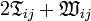$~2 \mathfrak{T}_{ij} + \mathfrak{W}_{ij}$$~=$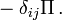$~- \delta_{ij}\Pi \, .$

[This] provides six integral relations which must obtain whenever the conditions are stationary.

When viewing the (generally ellipsoidal) configuration from a rotating frame of reference, the 2nd-order TVE takes on the more general form: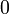$~0$$~=$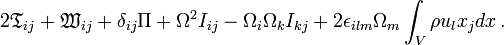$~ 2 \mathfrak{T}_{ij} + \mathfrak{W}_{ij} + \delta_{ij}\Pi + \Omega^2 I_{ij} - \Omega_i\Omega_k I_{kj} + 2\epsilon_{ilm}\Omega_m \int_V \rho u_lx_j dx \, .$ [ EFE, Chapter 2, §12, Eq. (64) ]

EFE (p. 57) also shows that … The potential energy tensor … for a homogeneous ellipsoid is given by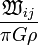$~\frac{\mathfrak{W}_{ij}}{\pi G\rho}$$~=$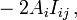$~-2A_i I_{ij} \, ,$ [ EFE, Chapter 3, §22, Eq. (128) ]

where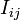$~I_{ij}$$~=$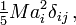$~\tfrac{1}{5} Ma_i^2 \delta_{ij} \, ,$ [ EFE, Chapter 3, §22, Eq. (129) ]

is the moment of inertia tensor.

EFE (p. 130) states that … The kinematical requirement, that the motion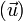$~(\vec{u})$, associated with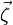$~\vec{\zeta}$, preserves the ellipsoidal boundary, leads to the following expressions for its components: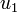$~u_1$$~=$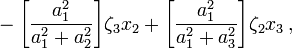$~- \biggl[ \frac{a_1^2}{a_1^2 + a_2^2}\biggr] \zeta_3 x_2 + \biggl[ \frac{a_1^2}{a_1^2+a_3^2}\biggr] \zeta_2 x_3 \, ,$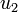$~u_2$$~=$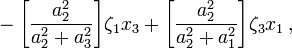$~- \biggl[ \frac{a_2^2}{a_2^2 + a_3^2}\biggr] \zeta_1 x_3 + \biggl[ \frac{a_2^2}{a_2^2+a_1^2}\biggr] \zeta_3 x_1 \, ,$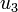$~u_3$$~=$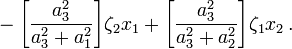$~- \biggl[ \frac{a_3^2}{a_3^2 + a_1^2}\biggr] \zeta_2 x_1 + \biggl[ \frac{a_3^2}{a_3^2+a_2^2}\biggr] \zeta_1 x_2 \, .$ [ EFE, Chapter 7, §47, Eq. (1) ]

## General Coefficient Expressions

As has been detailed in an accompanying chapter, the gravitational potential anywhere inside or on the surface,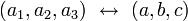$~(a_1,a_2,a_3) ~\leftrightarrow~(a,b,c)$, of an homogeneous ellipsoid may be given analytically in terms of the following three coefficient expressions: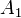$~A_1$$~=$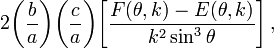$~2\biggl(\frac{b}{a}\biggr)\biggl(\frac{c}{a}\biggr) \biggl[ \frac{F(\theta,k) - E(\theta,k)}{k^2 \sin^3\theta} \biggr] \, ,$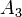$~A_3$$~=$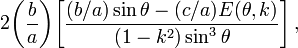$~2\biggl(\frac{b}{a}\biggr) \biggl[ \frac{(b/a) \sin\theta - (c/a)E(\theta,k)}{(1-k^2) \sin^3\theta} \biggr] \, ,$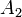$~A_2$$~=$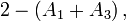$~2 - (A_1+A_3) \, ,$

where,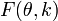$~F(\theta,k)$ and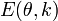$~E(\theta,k)$ are incomplete elliptic integrals of the first and second kind, respectively, with arguments,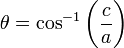$~\theta = \cos^{-1} \biggl(\frac{c}{a} \biggr)$ and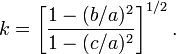$~k = \biggl[\frac{1 - (b/a)^2}{1 - (c/a)^2} \biggr]^{1/2} \, .$ [ EFE, Chapter 3, §17, Eq. (32) ]

Describe …

Describe …

Describe …